MCAT Physical : General Principles and Properties

Example Questions

← Previous 1 3

Example Question #161 : Mcat Physical Sciences

At a local concert, a speaker is set up to produce low-pitched base sounds with a frequency range of 20Hz to 200Hz, which can be modeled as  sine waves. In a simplified model, the sound waves the speaker produces can be modeled as a cylindrical pipe with one end closed that travel through the air at a velocity of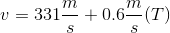, where T is the temperature in °C.

What is the wavelength of a 150Hz beat at 20ºC?

4.2m

3.1m

1.7m

2.3m

2.3m

Explanation:

This question is asking us to determine the wavelength of a sound wave at a specific temperature. From the equation presented to us in the paragraph above, we can calculate the wave velocity.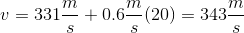Now, we can use this velocity to calculate the wavelength.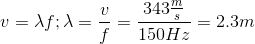Example Question #162 : Mcat Physical Sciences

A stretched string of length L, mass M, and tension T is vibrating at its fundamental frequency. Which of the following changes takes place if the vibration frequency of the string increases, but tension and mass density remain constant?

Wavelength decreases

Velocity increases

None of the other options

More than one of the other options

Number of nodes decreases

Wavelength decreases

Explanation:

We can use the equation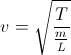together with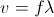. If T is constant, v cannot change assuming the mass density, m/L, is constant. Thus,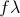must be constant; if f increases,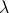must decrease.

Example Question #163 : Mcat Physical Sciences

What is the wavelength of a sound traveling at a frequency of 3000Hz?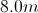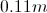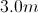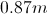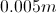Explanation:

The wavelength of a sound can be found by utilizing the equation,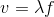. where v is the velocity of sound,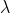is the wavelength, and f is the frequency. You should know that sound normally travels with a speed of 340m/s, unless otherwise stated. With the information given we can find the wavelength of the traveling sound to be 0.11m.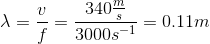Example Question #164 : Mcat Physical Sciences

Picture a transverse wave traveling through water. After the crest of one wave hits a stationary object in the water, an observer counts eight more crests hitting the same object in fifteen seconds. The frequency of the waves is __________.

120s

1.9s

1.9s-1

0.53s-1

0.53s-1

Explanation:

Right away you can rule out the answers with units in seconds, as the unit of frequency is an inverse second, or Hz. Frequency is measured in cycles per second. If eight crests pass a given point in fifteen seconds, the frequency is given by the number of crests divided by the time period.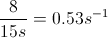Example Question #165 : Mcat Physical Sciences

A transverse wave has a velocity of 5.2m/s. If ten cycles pass a given point in 1.6s, what are the wave’s period and wavelength?

6.25s and 8.3m

0.16s and 0.83m

0.16s and 8.3m

6.25s and 0.83m

0.16s and 0.83m

Explanation:

First calculate the frequency of the wave (cycles/sec). The problem tells us that there are ten cycles in 1.6s.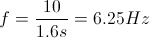Next find the period by taking the inverse of the frequency.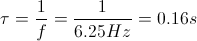Finally, find the wavelength by dividing the velocity by the frequency.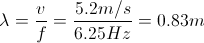Example Question #166 : Mcat Physical Sciences

An incandescent light bulb is shown through a glass prism. The certain wavlength of the light is then directed into a glass cuvette containing an unknown concentration of protein. Commonly, this process is called spectroscopy and is used to determine the concentrations of DNA, RNA, and proteins in solutions. The indices of reflection of air, glass, and the solution are 1, 1.5, and 1.3, respectively.

What property of light does not change when it enters the prism?

Velocity

More than one of these stays constant

Frequency

Wavelength

Frequency

Explanation:

The frequency of light does not change when it enters a medium with a different index of refraction; in this case, that new medium is the glass of the prism. From the velocity of light equation we know the relationship between velocity and frequency.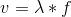v is the velocity of light,is the wavelength, and f is the frequency. When light enters the prism, its velocity changes due to the new index of refraction, but its frequency remains constant.

Because the frequency does not change, we can see that velocity is directly proportional to wavelength; thus, the shorter the wavelength, the slower the velocity. So both wavelength and velocity change when frequency is constant.

Example Question #167 : Mcat Physical Sciences

What is the relationship between frequency and period of a sine wave?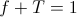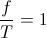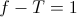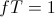Explanation:

The period of a wave is equal to the reciprocal of the frequency: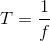Respectively, frequency is the reciprocal of period. By definition, the product of two reciprocals is one.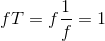Example Question #168 : Mcat Physical Sciences

Waves hit a beach every three seconds. The horizontal distance between an adjacent maximum and minimum is one meter. What is the speed of the waves?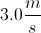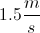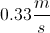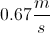Explanation:

Wave velocity is given by the product of frequency and wavelength: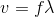In the question, we are given the period (waves per second). To find the frequency, we will need to take the reciprocal of the period.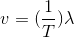Using the values given in the question, we can find the velocity of the waves. The wavelength is twice the distance between adjacent maxima and minima, making our wavelength two meters.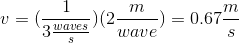Example Question #169 : Mcat Physical Sciences

An electron falls from an excited state to its ground state, emitting a photon at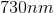. What is the frequency of the emitted light?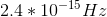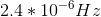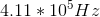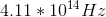Explanation:

The relationship between wavelength and frequency is given by the equation:In this case, the velocity will be equal to the speed of light.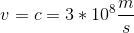Using this value and the given wavelength, we can find the frequency of the photon. Keep in mind that the wavelength must be given in meters.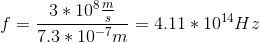Example Question #170 : Mcat Physical Sciences

What are the frequency and wavelength of a sound wave with a period of 0.04s and a velocity of 575m/s?

25Hz and 14,400m

25Hz and 0.44m

25Hz and 23m

1.0Hz and 14,400m

25Hz and 23m

Explanation:

Solve for frequency by taking the inverse of the period.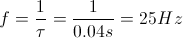Next, solve for wavelength by dividing velocity by frequency.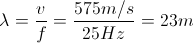← Previous 1 3